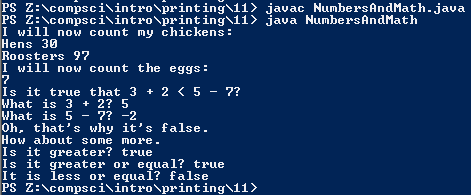# Assignemnt #11, Numbers and Math

## Code

```      /// Name: Alex Pinder
/// Period: 5
/// Program Name: Numbers and Math
/// File Name: NumbersAndMath.java
/// Date Finished: 9/11/2015

public class NumbersAndMath
{
public static void main( String[] args )
{
// This prints "I will now count my chickens:"
System.out.println( "I will now count my chickens:" );

// This does the math in the parentheses and displays it after "Hens " and "Roosters " respectively
System.out.println( "Hens " + ( 25 + 30 / 6 ) );
System.out.println( "Roosters " + ( 100 - 25 * 3 % 4 ) );

// This line prints "I will now count the eggs:"
System.out.println( "I will now count the eggs:" );

// This line prints the answer to the equation in the parentheses
System.out.println( ( 3 + 2 + 1 - 5 + 4 % 2 - 1 / 4 + 6 ) );
// This prints "Is it true that 3 + 2 < 5 - 7?"
System.out.println( "Is it true that 3 + 2 < 5 - 7?" );

// This prints the answers to 3 + 2 and 5 - 7 respectively
System.out.println( "What is 3 + 2? " + ( 3 + 2 ) );
System.out.println( "What is 5 - 7? " + ( 5 - 7 ) );

// This prints "Oh, thats's why it's false."
System.out.println( "Oh, that's why it's false." );

// This prints "How about some more"
System.out.println( "How about some more." );

// These say weather the equation in the parentheses is true or false
System.out.println( "Is it greater? " + ( 5 > -2 ) );
System.out.println( "Is it greater or equal? " + ( 5 >= -2 ) );
System.out.println( "It is less or equal? " + ( 5 <= -2 ) );
}
}
```

### Picture of the output